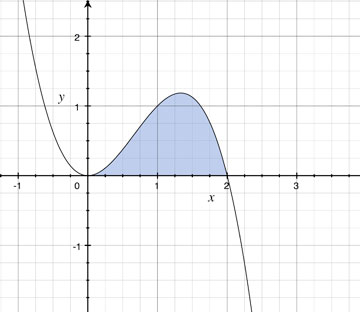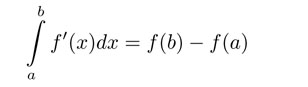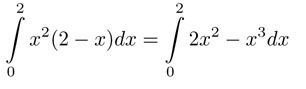SEARCH HOMEMath Central Quandaries & QueriesQuestion from Morag, a parent: y=(x-1)(x-2)(x-3) find the area bounded by this curve and the x-axis between x=1 and x=2 What is the area bounded by the curve y=X2(2-X) and the x axis between x=0 and x=2?Hi Morag,

Let's just look at the last one since both can be solved the same way. First let's look at the described area you are trying to findIn math whenever someone is trying to find the area of a curve they use an integral. In simple terms an integral is the area of the region by the graph of ƒ, the x-axis, and the vertical lines x = a and x = b. It can be written asSo for your equation (it makes the question easier if you distribute):Integrals are also known as anti-derivatives (the opposite of derivatives). If f'(x)=x2(2-x), what could f(x) be? Once f(x) is determined, find f(2)-f(0).

Hope this helps,

JaniceMath Central is supported by the University of Regina and The Pacific Institute for the Mathematical Sciences.